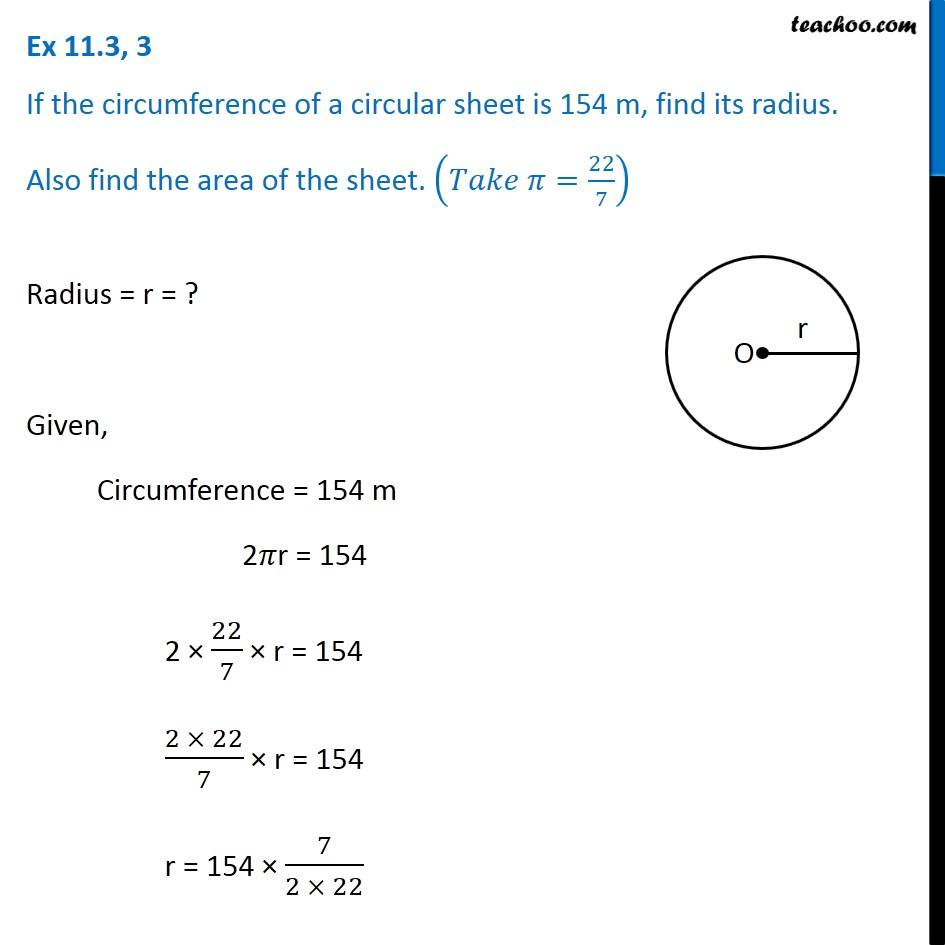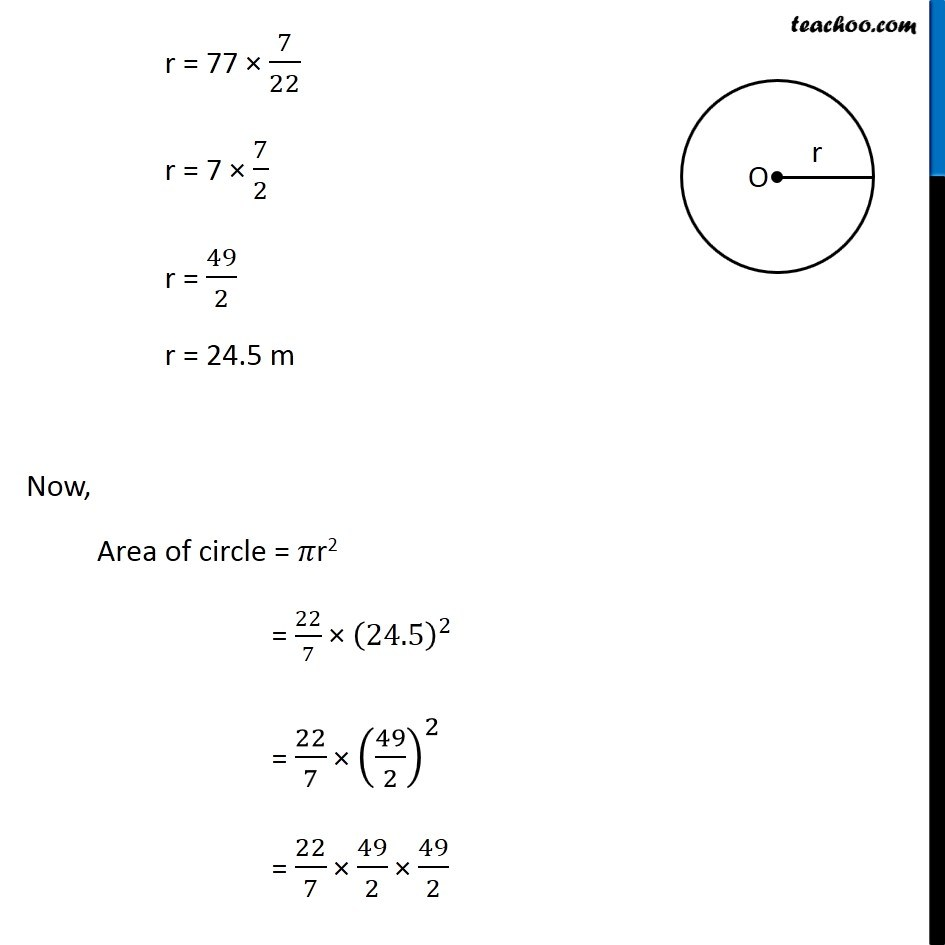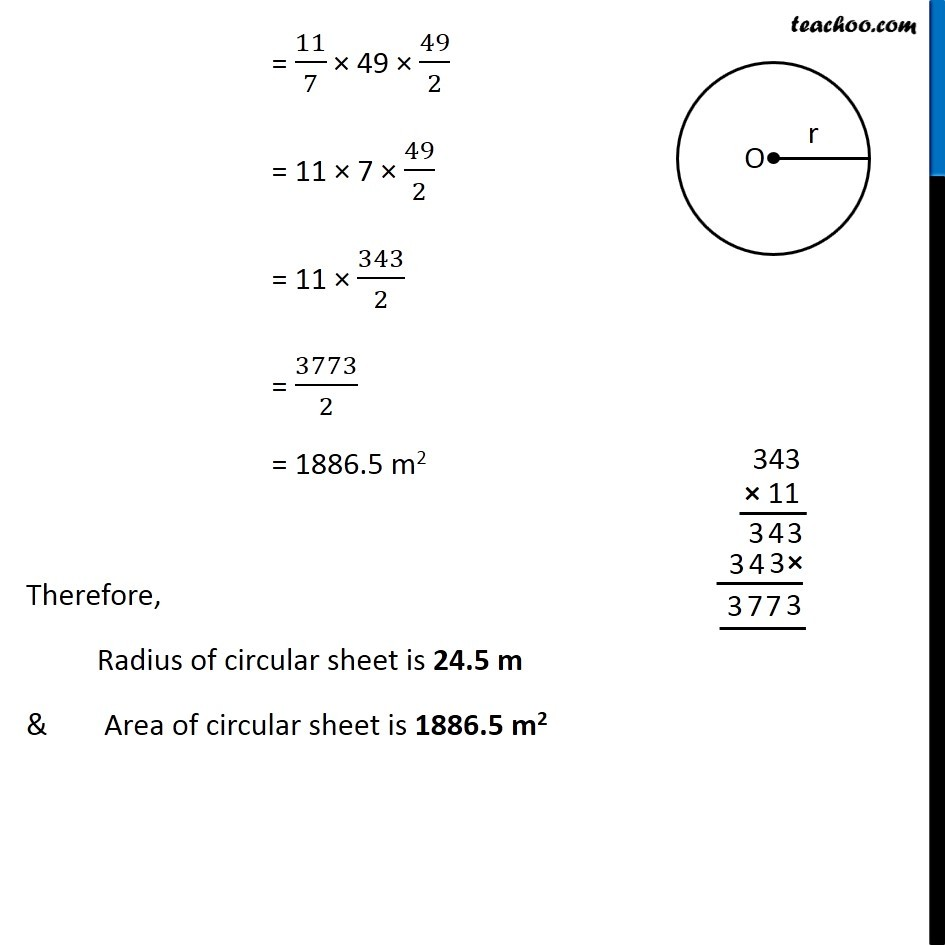Area of circle

Chapter 9 Class 7 Perimeter and Area
Concept wiseGet live Maths 1-on-1 Classs - Class 6 to 12

### Transcript

Ex 9.2, 3 If the circumference of a circular sheet is 154 m, find its radius. Also find the area of the sheet. (𝑇𝑎𝑘𝑒 𝜋=22/7) Radius = r = ? Given, Circumference = 154 m 2𝜋r = 154 2 × 22/7 × r = 154 (2 × 22)/7 × r = 154 r = 154 × 7/(2 × 22) r = 77 × 7/22 r = 7 × 7/2 r = 49/2 r = 24.5 m Now, Area of circle = 𝜋r2 = 22/7 × (24.5)^2 = 22/7 × (49/2)^2 = 22/7 × 49/2 × 49/2 = 11/7 × 49 × 49/2 = 11 × 7 × 49/2 = 11 × 343/2 = 3773/2 = 1886.5 m2 Therefore, Radius of circular sheet is 24.5 m & Area of circular sheet is 1886.5 m2